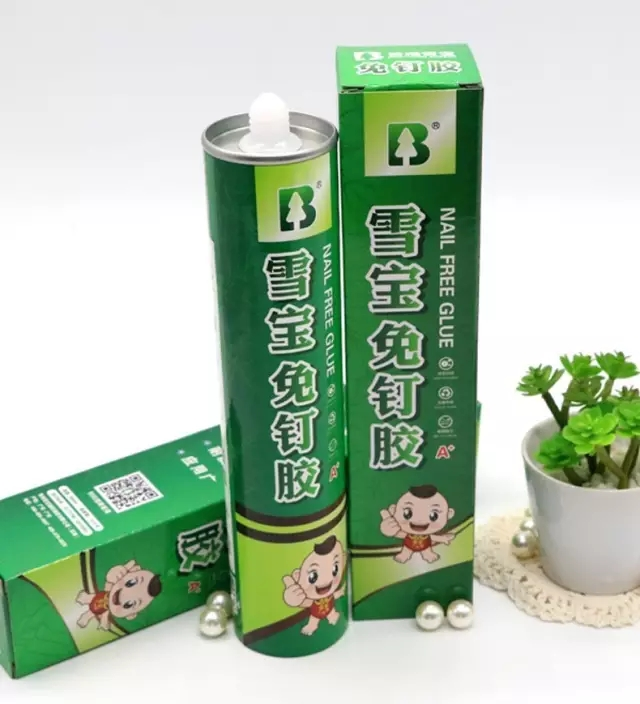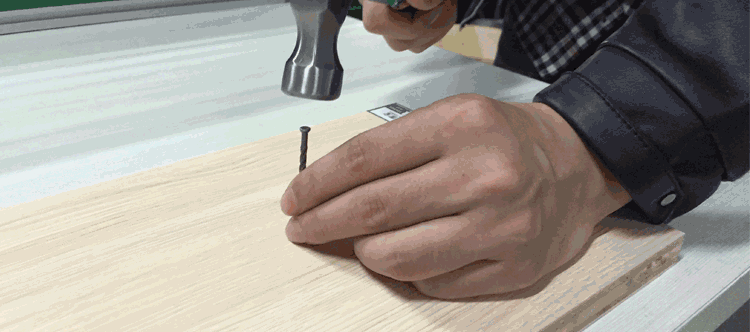<blockquote class="yead_editor" data-author="Wxeditor'" helvitica="" neue',="" helvitica,="" arial,="" 'hiragino="" sans="" gb',="" 'microsoft="" yahei',="" 'arial="" regular',="" jhenghei',="" sans-serif;="" font-size:="" 14px;="" line-height:="" normal;="" white-space:="" border:="" 0px;="" padding-left:="" margin:="" 5px="" auto;"="">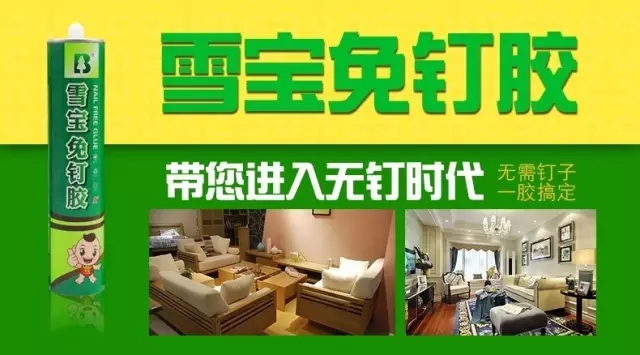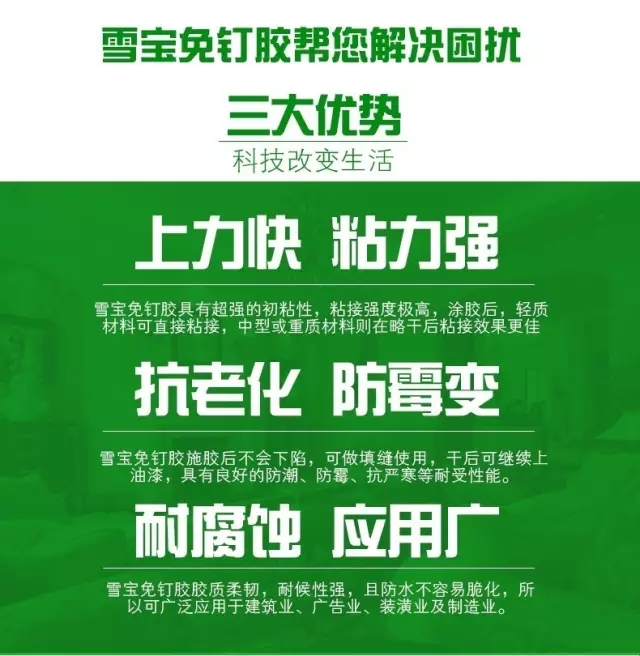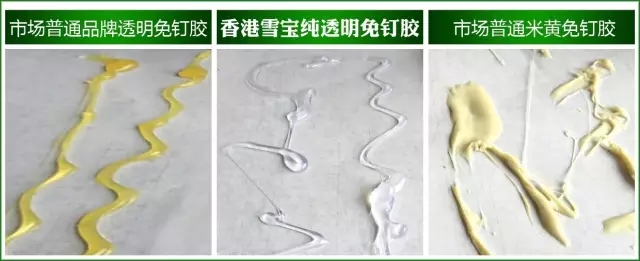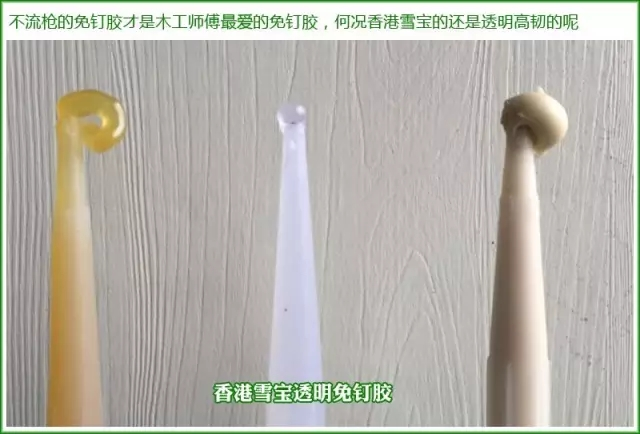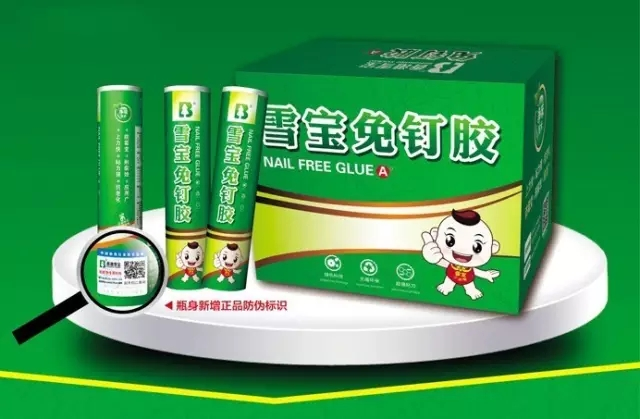1

2

3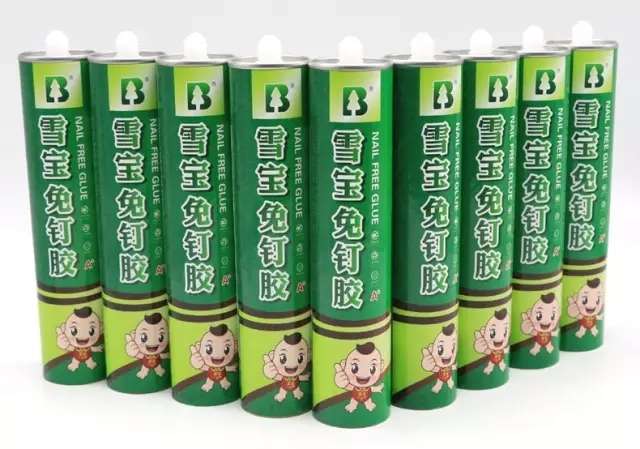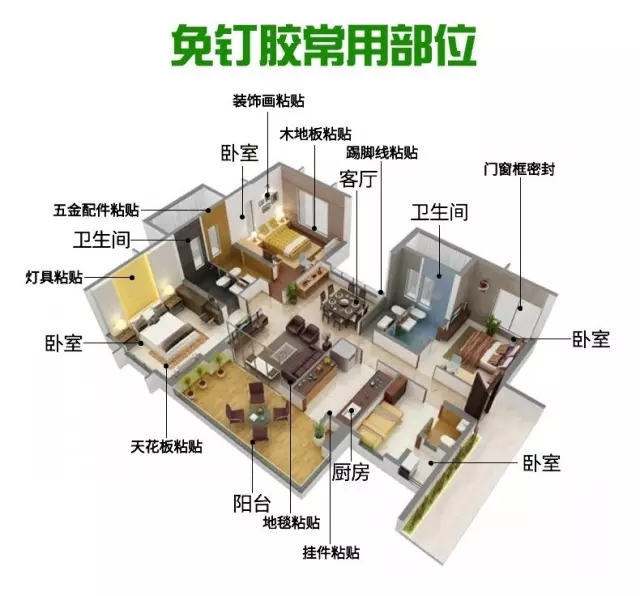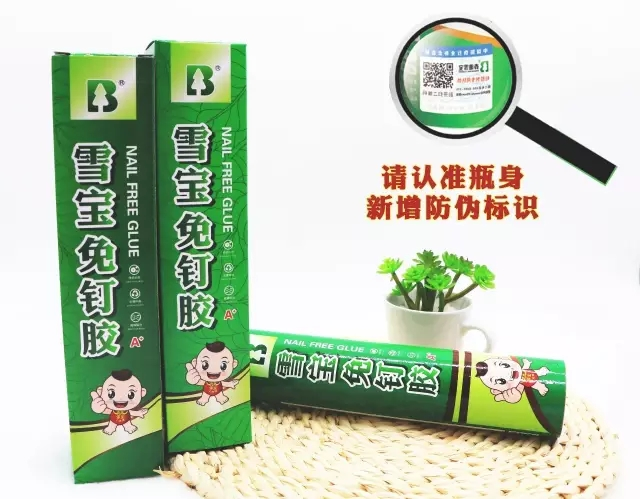“免”说明了它的存在状态

“钉”说明了它的性质。